Main MRPT website > C++ reference for MRPT 1.5.7
mrpt::math::CMatrix Class Reference

## Detailed Description

This class is a "CSerializable" wrapper for "CMatrixFloat".

Note
For a complete introduction to Matrices and vectors in MRPT, see: http://www.mrpt.org/Matrices_vectors_arrays_and_Linear_Algebra_MRPT_and_Eigen_classes

Definition at line 30 of file CMatrix.h.

#include <mrpt/math/CMatrix.h>

Inheritance diagram for mrpt::math::CMatrix: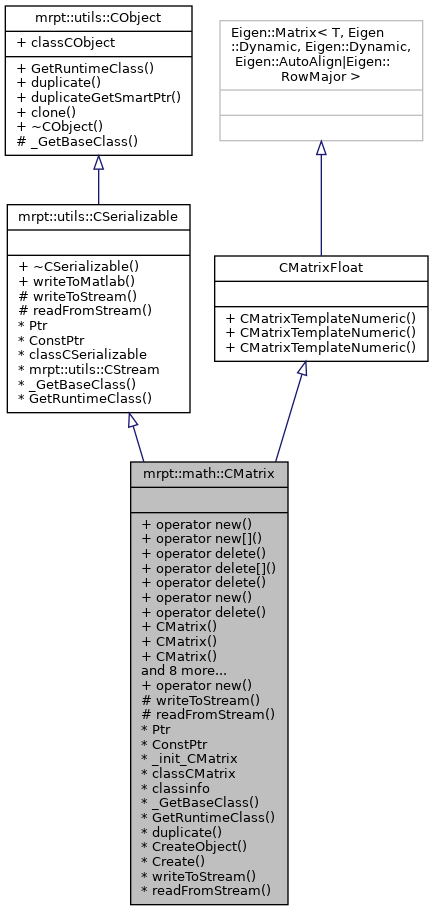## Public Types

typedef Eigen::Matrix< T, Eigen::Dynamic, Eigen::Dynamic, Eigen::AutoAlign|Eigen::RowMajor > Base

typedef CMatrixTemplateNumeric< T > mrpt_autotype

## Public Member Functions

voidoperator new (size_t size)

voidoperator new[] (size_t size)

void operator delete (void *ptr) throw ()

void operator delete[] (void *ptr) throw ()

void operator delete (void *memory, void *ptr) throw ()

voidoperator new (size_t size, const std::nothrow_t &) throw ()

void operator delete (void *ptr, const std::nothrow_t &) throw ()

CMatrix ()
Constructor. More...

CMatrix (size_t row, size_t col)
Constructor. More...

CMatrix (const CMatrixFloat &m)
Copy constructor. More...

CMatrix (const CMatrixTemplateNumeric< double > &m)
Copy constructor. More...

CMatrix (const TPose2D &p)
Constructor from a TPose2D, which generates a 3x1 matrix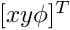. More...

CMatrix (const TPose3D &p)
Constructor from a mrpt::poses::CPose6D, which generates a 6x1 matrix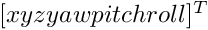. More...

CMatrix (const TPoint2D &p)
Constructor from a TPoint2D, which generates a 2x1 matrix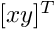. More...

CMatrix (const TPoint3D &p)
Constructor from a TPoint3D, which generates a 3x1 matrix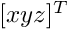. More...

template<class OTHERMAT >
CMatrixoperator= (const OTHERMAT &m)
Assignment operator for float matrixes. More...

template<typename OtherDerived >
CMatrixoperator= (const Eigen::MatrixBase< OtherDerived > &other)

template<typename OtherDerived >
CMatrix (const Eigen::MatrixBase< OtherDerived > &other)

virtual mxArraywriteToMatlab () const
Introduces a pure virtual method responsible for writing to a mxArray Matlab object, typically a MATLAB struct whose contents are documented in each derived class. More...

mrpt::utils::CObjectPtr duplicateGetSmartPtr () const
Returns a copy of the object, indepently of its class, as a smart pointer (the newly created object will exist as long as any copy of this smart pointer). More...

CObjectclone () const
Cloning interface for smart pointers. More...

## Static Public Member Functions

static voidoperator new (size_t size, void *ptr)

## Static Public Attributes

static const mrpt::utils::TRuntimeClassId classCObject

RTTI stuff
static const mrpt::utils::TRuntimeClassId classCSerializable

## Protected Member Functions

CSerializable virtual methods
void writeToStream (mrpt::utils::CStream &out, int *getVersion) const
Introduces a pure virtual method responsible for writing to a CStream. More...

void readFromStream (mrpt::utils::CStream &in, int version)
Introduces a pure virtual method responsible for loading from a CStream This can not be used directly be users, instead use "stream >> object;" for reading it from a stream or "stream >> object_ptr;" if the class is unknown apriori. More...

## RTTI stuff

typedef CMatrixPtr Ptr

typedef CMatrixPtr ConstPtr

static mrpt::utils::CLASSINIT _init_CMatrix

static mrpt::utils::TRuntimeClassId classCMatrix

static const mrpt::utils::TRuntimeClassIdclassinfo

static const mrpt::utils::TRuntimeClassId_GetBaseClass ()

virtual const mrpt::utils::TRuntimeClassIdGetRuntimeClass () const
Returns information about the class of an object in runtime. More...

virtual mrpt::utils::CObjectduplicate () const
Returns a copy of the object, indepently of its class. More...

static mrpt::utils::CObjectCreateObject ()

static CMatrixPtr Create ()

## ◆ Base

template<class T>
 typedef Eigen::Matrix mrpt::math::CMatrixTemplateNumeric< T >::Base
inherited

Definition at line 42 of file CMatrixTemplateNumeric.h.

## ◆ ConstPtr

 typedef CMatrixPtr mrpt::math::CMatrix::ConstPtr

Definition at line 33 of file CMatrix.h.

## ◆ mrpt_autotype

template<class T>
 typedef CMatrixTemplateNumeric mrpt::math::CMatrixTemplateNumeric< T >::mrpt_autotype
inherited

Definition at line 43 of file CMatrixTemplateNumeric.h.

## ◆ Ptr

 typedef CMatrixPtr mrpt::math::CMatrix::Ptr

A typedef for the associated smart pointer

Definition at line 33 of file CMatrix.h.

## ◆ CMatrix() [1/9]

 mrpt::math::CMatrix::CMatrix ( )
inline

Constructor.

Definition at line 37 of file CMatrix.h.

## ◆ CMatrix() [2/9]

 mrpt::math::CMatrix::CMatrix ( size_t row, size_t col )
inline

Constructor.

Definition at line 41 of file CMatrix.h.

## ◆ CMatrix() [3/9]

 mrpt::math::CMatrix::CMatrix ( const CMatrixFloat & m )
inline

Copy constructor.

Definition at line 46 of file CMatrix.h.

## ◆ CMatrix() [4/9]

 mrpt::math::CMatrix::CMatrix ( const CMatrixTemplateNumeric< double > & m )
inline

Copy constructor.

Definition at line 51 of file CMatrix.h.

## ◆ CMatrix() [5/9]

 CMatrix::CMatrix ( const TPose2D & p )
explicit

Constructor from a TPose2D, which generates a 3x1 matrix.

Definition at line 27 of file CMatrix.cpp.

## ◆ CMatrix() [6/9]

 CMatrix::CMatrix ( const TPose3D & p )
explicit

Constructor from a mrpt::poses::CPose6D, which generates a 6x1 matrix.

Definition at line 29 of file CMatrix.cpp.

## ◆ CMatrix() [7/9]

 CMatrix::CMatrix ( const TPoint2D & p )
explicit

Constructor from a TPoint2D, which generates a 2x1 matrix.

Definition at line 31 of file CMatrix.cpp.

## ◆ CMatrix() [8/9]

 CMatrix::CMatrix ( const TPoint3D & p )
explicit

Constructor from a TPoint3D, which generates a 3x1 matrix.

Definition at line 33 of file CMatrix.cpp.

## ◆ CMatrix() [9/9]

template<typename OtherDerived >
 mrpt::math::CMatrix::CMatrix ( const Eigen::MatrixBase< OtherDerived > & other )
inline

Constructor from any other Eigen class

Definition at line 82 of file CMatrix.h.

## ◆ _GetBaseClass()

 static const mrpt::utils::TRuntimeClassId* mrpt::math::CMatrix::_GetBaseClass ( )
staticprotected

## ◆ clone()

 CObject* mrpt::utils::CObject::clone ( ) const
inlineinherited

Cloning interface for smart pointers.

Definition at line 143 of file CObject.h.

## ◆ Create()

 static CMatrixPtr mrpt::math::CMatrix::Create ( )
static

## ◆ CreateObject()

 static mrpt::utils::CObject* mrpt::math::CMatrix::CreateObject ( )
static

## ◆ duplicate()

 virtual mrpt::utils::CObject* mrpt::math::CMatrix::duplicate ( ) const
virtual

Returns a copy of the object, indepently of its class.

Implements mrpt::utils::CObject.

## ◆ duplicateGetSmartPtr()

 mrpt::utils::CObjectPtr mrpt::utils::CObject::duplicateGetSmartPtr ( ) const
inlineinherited

Returns a copy of the object, indepently of its class, as a smart pointer (the newly created object will exist as long as any copy of this smart pointer).

Definition at line 140 of file CObject.h.

## ◆ GetRuntimeClass()

 virtual const mrpt::utils::TRuntimeClassId* mrpt::math::CMatrix::GetRuntimeClass ( ) const
virtual

Returns information about the class of an object in runtime.

Reimplemented from mrpt::utils::CSerializable.

## ◆ operator delete() [1/3]

 void mrpt::math::CMatrix::operator delete ( void * ptr ) throw ( )
inline

Definition at line 33 of file CMatrix.h.

## ◆ operator delete() [2/3]

 void mrpt::math::CMatrix::operator delete ( void * memory, void * ptr ) throw ( )
inline

Definition at line 33 of file CMatrix.h.

## ◆ operator delete() [3/3]

 void mrpt::math::CMatrix::operator delete ( void * ptr, const std::nothrow_t & ) throw ( )
inline

Definition at line 33 of file CMatrix.h.

## ◆ operator delete[]()

 void mrpt::math::CMatrix::operator delete[] ( void * ptr ) throw ( )
inline

Definition at line 33 of file CMatrix.h.

## ◆ operator new() [1/3]

 void* mrpt::math::CMatrix::operator new ( size_t size )
inline

Definition at line 33 of file CMatrix.h.

## ◆ operator new() [2/3]

 static void* mrpt::math::CMatrix::operator new ( size_t size, void * ptr )
inlinestatic

Definition at line 33 of file CMatrix.h.

## ◆ operator new() [3/3]

 void* mrpt::math::CMatrix::operator new ( size_t size, const std::nothrow_t & ) throw ( )
inline

Definition at line 33 of file CMatrix.h.

## ◆ operator new[]()

 void* mrpt::math::CMatrix::operator new[] ( size_t size )
inline

Definition at line 33 of file CMatrix.h.

## ◆ operator=() [1/2]

template<class OTHERMAT >
 CMatrix& mrpt::math::CMatrix::operator= ( const OTHERMAT & m )
inline

Assignment operator for float matrixes.

Definition at line 68 of file CMatrix.h.

## ◆ operator=() [2/2]

template<typename OtherDerived >
 CMatrix& mrpt::math::CMatrix::operator= ( const Eigen::MatrixBase< OtherDerived > & other )
inline

Assignment operator from any other Eigen class

Definition at line 76 of file CMatrix.h.

 void CMatrix::readFromStream ( mrpt::utils::CStream & in, int version )
protectedvirtual

Introduces a pure virtual method responsible for loading from a CStream This can not be used directly be users, instead use "stream >> object;" for reading it from a stream or "stream >> object_ptr;" if the class is unknown apriori.

Parameters
 in The input binary stream where the object data must read from. version The version of the object stored in the stream: use this version number in your code to know how to read the incoming data.
Exceptions
 std::exception On any error, see CStream::ReadBuffer
CStream

Implements mrpt::utils::CSerializable.

Definition at line 57 of file CMatrix.cpp.

References MRPT_THROW_UNKNOWN_SERIALIZATION_VERSION, setSize(), and version.

## ◆ writeToMatlab()

 virtual mxArray* mrpt::utils::CSerializable::writeToMatlab ( ) const
inlinevirtualinherited

Introduces a pure virtual method responsible for writing to a mxArray Matlab object, typically a MATLAB struct whose contents are documented in each derived class.

Returns
A new mxArray (caller is responsible of memory freeing) or NULL is class does not support conversion to MATLAB.

Definition at line 79 of file CSerializable.h.

## ◆ writeToStream()

 void CMatrix::writeToStream ( mrpt::utils::CStream & out, int * getVersion ) const
protectedvirtual

Introduces a pure virtual method responsible for writing to a CStream.

This can not be used directly be users, instead use "stream << object;" for writing it to a stream.

Parameters
 out The output binary stream where object must be dumped. getVersion If NULL, the object must be dumped. If not, only the version of the object dump must be returned in this pointer. This enables the versioning of objects dumping and backward compatibility with previously stored data.
Exceptions
 std::exception On any error, see CStream::WriteBuffer
CStream

Implements mrpt::utils::CSerializable.

Definition at line 38 of file CMatrix.cpp.

## ◆ _init_CMatrix

 mrpt::utils::CLASSINIT mrpt::math::CMatrix::_init_CMatrix
staticprotected

Definition at line 33 of file CMatrix.h.

## ◆ classCMatrix

 mrpt::utils::TRuntimeClassId mrpt::math::CMatrix::classCMatrix
static

Definition at line 33 of file CMatrix.h.

## ◆ classCObject

 const mrpt::utils::TRuntimeClassId mrpt::utils::CObject::classCObject
staticinherited

Definition at line 128 of file CObject.h.

## ◆ classCSerializable

 const mrpt::utils::TRuntimeClassId mrpt::utils::CSerializable::classCSerializable
staticinherited

Definition at line 42 of file CSerializable.h.

## ◆ classinfo

 const mrpt::utils::TRuntimeClassId* mrpt::math::CMatrix::classinfo
static

Definition at line 33 of file CMatrix.h.

 Page generated by Doxygen 1.8.14 for MRPT 1.5.7 Git: 8277875f6 Mon Jun 11 02:47:32 2018 +0200 at lun oct 28 01:50:49 CET 2019